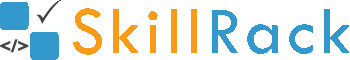Program to print Fibonacci series in Java2 years ago

In this article we will look at a Java program that will print Fibonacci series upto a certain number of terms using recursion.

The number of terms is passed as input.

import java.util.Scanner;

public class Hello {

public static void main(String[] args) {
Scanner sc = new Scanner(System.in);
int termsCount = sc.nextInt();
int sum = 1, curr = 1;
System.out.println(curr);
fibonacci(curr, sum, termsCount);
}

private static void fibonacci(int curr, int sum, int remainingTerms) {
if (remainingTerms != 1) {
System.out.print(sum);
fibonacci(sum, curr + sum, remainingTerms - 1);
}
}
}